基于时间序列LSTM模型的ENSO指数预报试验The Prediction of ENSO Indexes Based on Time Series LSTM Model

• 全文下载: PDF(3602KB)    PP.287-295   DOI: 10.12677/CCRL.2019.83032
• 下载量: 56  浏览量: 113   国家科技经费支持

To improve the prediction ability of El Niño-Southern Oscillation (ENSO), we use the Niño 3.4 and Southern Oscillation indexes during 1951-2008 to build time series long short-term memory (LSTM) model, and forecast that during 2009-2018. The analysis shows the time series LSTM model has a good forecasting ability for Niño 3.4 index in advance of 1 - 12 months, though underestimates the amplitude of the super El Niño event during 15/16 year. While time series LSTM model can only predict the SOI in advance of 1 - 3 months to a certain extent, because of the high frequency seasonal variability in SOI index. Further experiment proves that the 7-month run-mean SOI is also can be predicted well by time series LSTM model, similar with the Niño 3.4 index. Comparing with other statistical forecasting models, the time series LSTM model is efficient and convenience with ad-vantages in ENSO prediction.

1. 引言

2. 模型介绍

$\begin{array}{l}{i}_{t}=\sigma \left({W}_{i}{x}_{t}+{U}_{i}{h}_{t-1}+{b}_{i}\right)\\ {f}_{t}=\sigma \left({W}_{f}{x}_{t}+{U}_{f}{h}_{t-1}+{b}_{f}\right)\\ {u}_{t}=\mathrm{tanh}\left({W}_{u}{x}_{t}+{U}_{u}{h}_{t-1}+{b}_{u}\right)\\ {o}_{t}=\sigma \left({W}_{o}{x}_{t}+{U}_{o}{h}_{t-1}+{b}_{o}\right)\\ {c}_{t}={f}_{t}\odot {c}_{t-1}+{i}_{t}{u}_{t}\\ {h}_{t}={o}_{t}\odot \mathrm{tanh}\left({c}_{t}\right)\end{array}$ (1)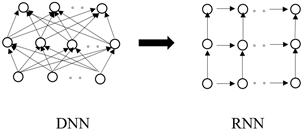Figure 1. The schematic diagram of DNN and RNN

3. 实验过程

3.1. 数据介绍

ENSO是一种发生在热带太平洋的海气相互作用过程，因此有许多定义从不同角度来描述了ENSO事件。在海洋中，最具代表性的描述是Niño 3.4指数，其定义是5˚N~5˚S，170˚W~120˚W区域海表面温度距平场的空间平均。而在大气中，最具有代表性的描述是南方涛动指数(Southern Oscillation index，简称SOI)，其定义为达尔文岛和塔希提岛标准化海平面气压异常场的差值(塔希提岛-达尔文岛)。因此，本文采用了由美国国家大气研究中心(National Center for Atmospheric Research，简称NCAR)提供的Niño 3.4指数，作为海洋中ENSO事件的指标，和来自美国海洋与大气中心(National Oceanic and Atmospheric Administration，简称NOAA)提供的SOI指数，作为大气中ENSO事件的衡量指标。两种指数的起止时间均为1951年1月至2018年12月。Figure 2. The mean (a), standard deviation (b), maximum (c), minimum (d) in train (red) and test (green) set of Niño 3.4 and SOI index

3.2. 模型训练

4. 结果分析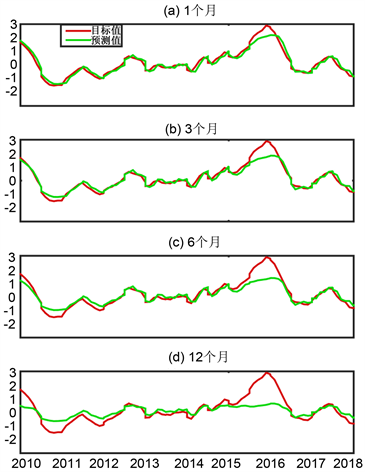Figure 3. Prediction results and observed Niño 3.4 index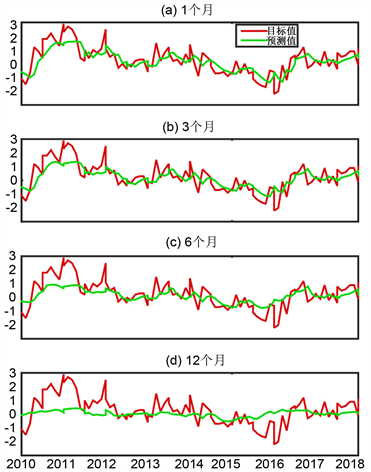Figure 4. Prediction results and observed SOI index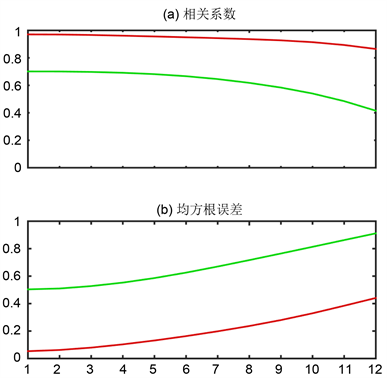Figure 5. The correlation coefficient and root-mean-square error between prediction results and observed Niño 3.4 index or SOIFigure 6. The power spectra density of Niño 3.4 (red) and SOI (green) index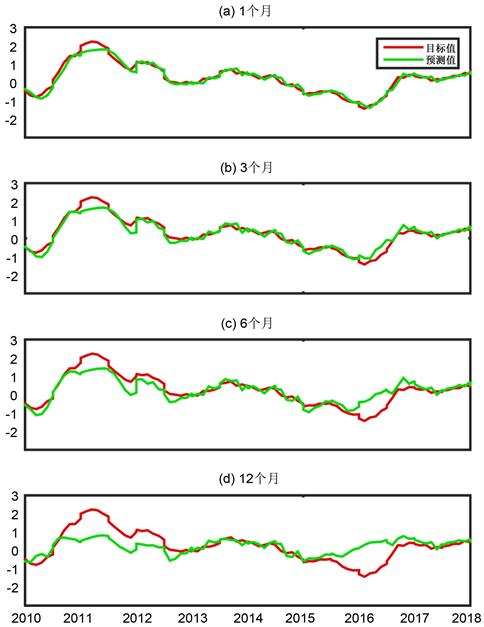Figure 7. Prediction results and observed 7-months run-mean SOI index

5. 结语

  Branston, A.G. and Vandendool, H.M. (1994) Long Lead Seasonal Forecasts—Where Do We Stand? Bulletin of the American Math-ematical Society, 75, 2097-2114. https://doi.org/10.1175/1520-0477(1994)075<2097:LLSFDW>2.0.CO;2  丁裕国, 程正泉, 程炳岩. MSSA-SVD典型回归模型及其用于ENSO预报的试验[J]. 气象学报, 2002, 60(3): 361-368.  刘科峰, 张军, 陈奕德, 等. 基于小波分解和支持向量机的ENSO预测试验[J]. 解放军理工大学学报: 自然科学版, 2011, 12(5): 531-535.  严军, 刘建文. 基于神经网路——奇异谱分析的ENSO指数预测[J]. 大气科学, 2005, 29(4): 620-626.  刘鑫达. 基于深度学习的气象温度预测研究[D]: [硕士学位论文]. 宁夏: 宁夏大学, 2016.  Feng, Q.Y., Vasile, R., Segond, M., et al. (2016) Climate Learn: A Machine-Learning Approach for Climate Prediction Using Network Measures. Geoscientific Model Development Discussions, 1-18.  Zhang, Q., Wang, H., Dong, J.Y., et al. (2017) Prediction of Sea Surface Temperature Using Long Short-Term Memory. IEEE Geoscience and Remote Sensing Letters, 14, 1745-1749. https://doi.org/10.1109/LGRS.2017.2733548  许柏宁, 姜金荣，郝卉群, 等. 一种基于区域海表面温度异常预测的ENSO预报深度学习模型[J]. 科研信息化技术和应用, 2017, 8(6): 65-76.  Hopfield, J.J. (1982) Neural Networks and Physical Systems with Emergent Collective Computational Abilities. Proceedings of the National Acade-my of Sciences, 79, 2554-2558. https://doi.org/10.1073/pnas.79.8.2554  Hochreiter, S. and Schmidhuber, J. (1997) Long Short-Term Memory. Neural Computation, 9, 1735-1780. https://doi.org/10.1162/neco.1997.9.8.1735  Cai, W., Santoso, A., Wang, G., et al. (2015) ENSO and Greenhouse Warming. Nature Climate Change, 5, 849-859. https://doi.org/10.1038/nclimate2743  Cai, W., Wang, G., Dewitte, B., et al. (2018) Increased Variability of Eastern Pacific El Niño under Greenhouse Warming. Nature, 564, 201-206. https://doi.org/10.1038/s41586-018-0776-9  刘秦玉, 谢尚平, 郑小童. 热带海洋–大气相互作用[M]. 北京: 高等教育出版社, 2013: 42-50.